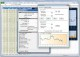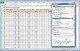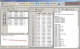Math & Scientific Tools software reviews

Showing 126 to 132 from 132 matchesNeuroXL Package 4.0.5 Math & Scientific Tools Neural network forecasting and data clustering tools for Microsoft Excel SharewareNeuroXL Predictor 4.0.5 Math & Scientific Tools NeuroXL Predictor is a neural network forecasting and add-in for Microsoft Excel SharewarePEEI 4.0.0.1 Math & Scientific Tools Solver of systems of partial differential equations. FreewarePTC Mathcad Express 3.1 Math & Scientific Tools PTC Mathcad Express is free-for-life engineering math software FreewareEngineering Power Tools Plus Edition 2.0.5 Math & Scientific Tools Engineering Power Tools Plus Edition solves common engineering problems quickly. SharewareMEPX 2018.04.04 Math & Scientific Tools MEPX - data analysis software for Windows, Mac OSX and Linux FreewareDreamCalc Scientific Graphing Calculator 5.0.4 Math & Scientific Tools A free scienfitic graphing calculator that's like using the real thing. Shareware